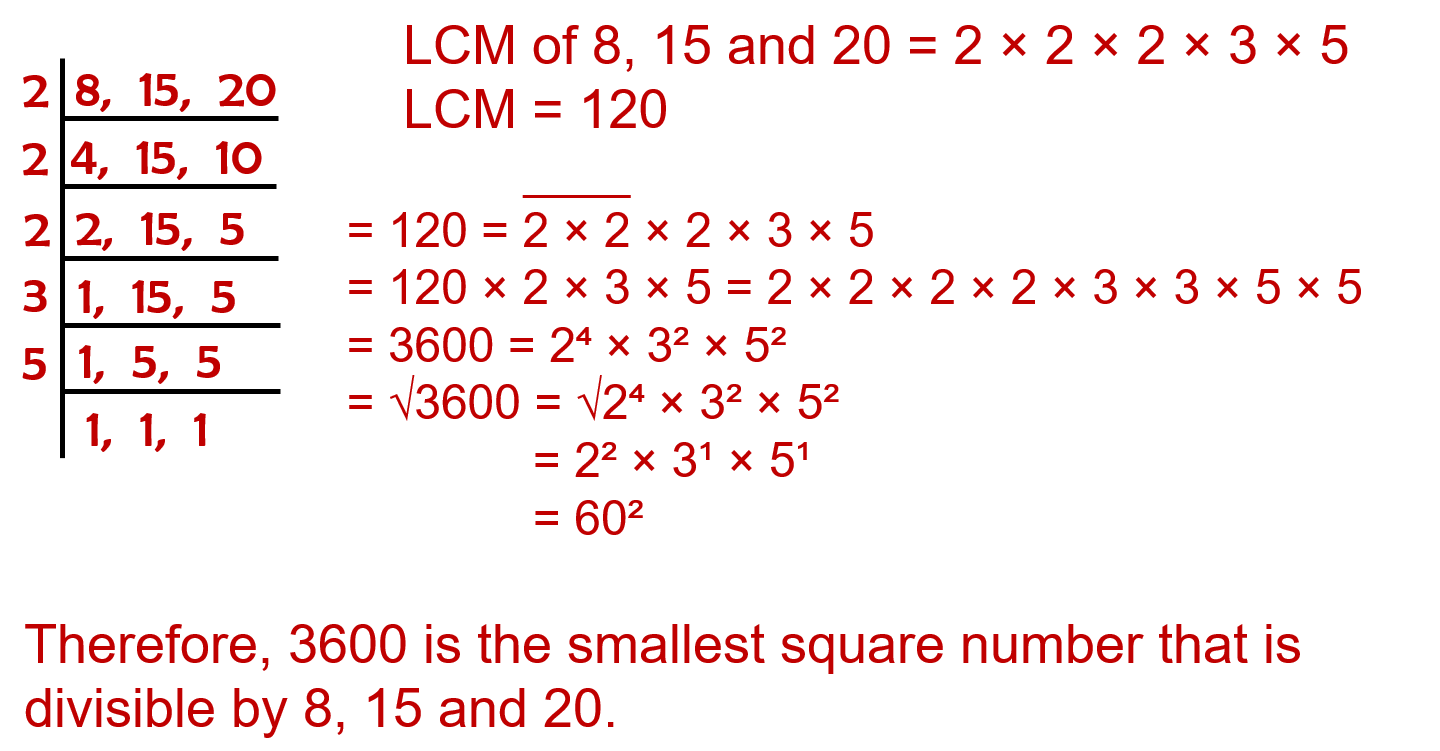# vs.eyeandcontacts.com

## Chapter 6 Squares and Square Roots Exercise 6.3

Question 1: What could be the possible ‘one’s’ digits of the square root of each of the following numbers?
i. 9801
ii. 99856
iii. 998001
iv. 657666025

i. The unit’s digit of 9801 is 1. If the number has the unit digit as 1, then its square root will end with 1, 9.

ii. The unit’s digit of 99856 is 6. If the number has the unit digit as 6, then its square root will end with 4, 6.

iii. The unit’s digit of 998001 is 1. If the number has the unit digit as 1, then its square root will end with 1, 9.

iv. The unit’s digit of 657666025 is 5. If the number has the unit digit as 5, then its square root will end with 5.

Question 2: Without doing any calculation, find the numbers which are surely not perfect squares.
i. 153
ii. 257
iii. 408
iv. 441
i. No, 153 is not a perfect square because its unit digit is 3.

ii. No, 257 is not a perfect square because its unit digit is 7.

iii. No, 408 is not a perfect square because its unit digit is 8.

iv. Yes, 441 is a perfect square because its unit digit is 1.

Question 3: Find the square roots of 100 and 169 by the method of repeated subtraction.Question 4: Find the square roots of the following numbers by the Prime Factorisation Method.
i. 729
ii. 400
iii. 1764
iv. 4096
v. 7744
vi. 9604
vii. 5929
viii. 9216
ix. 529
x. 8100

i.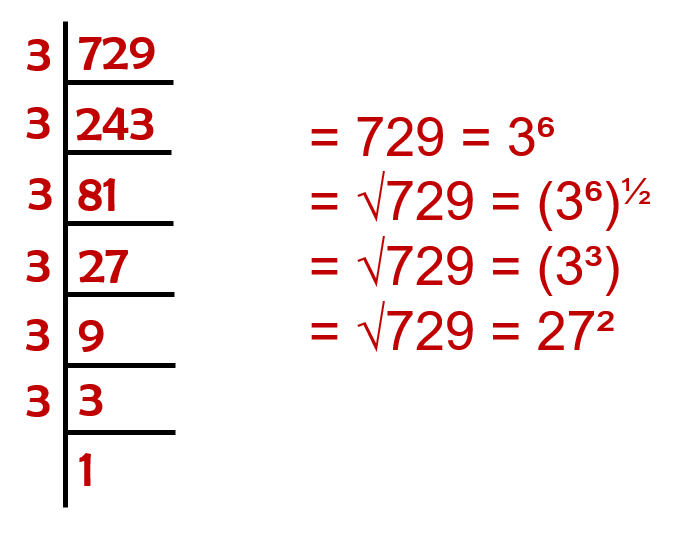ii.iii.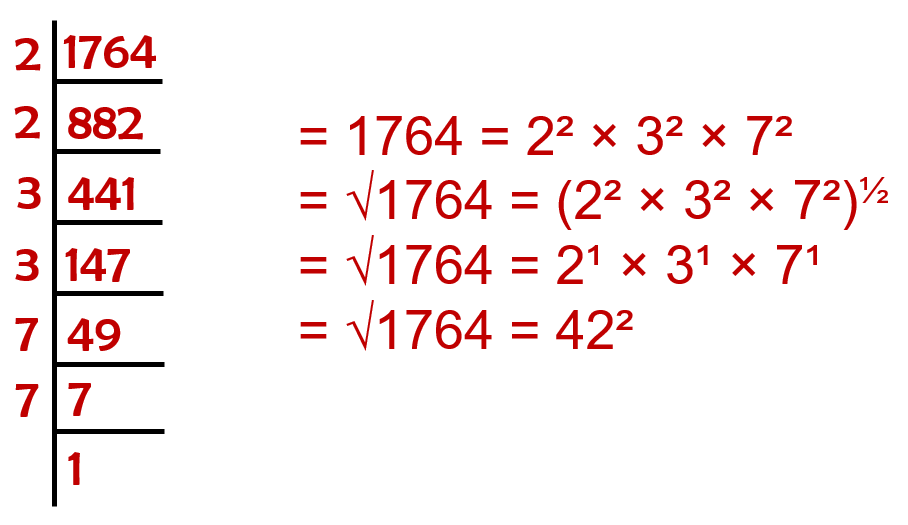iv.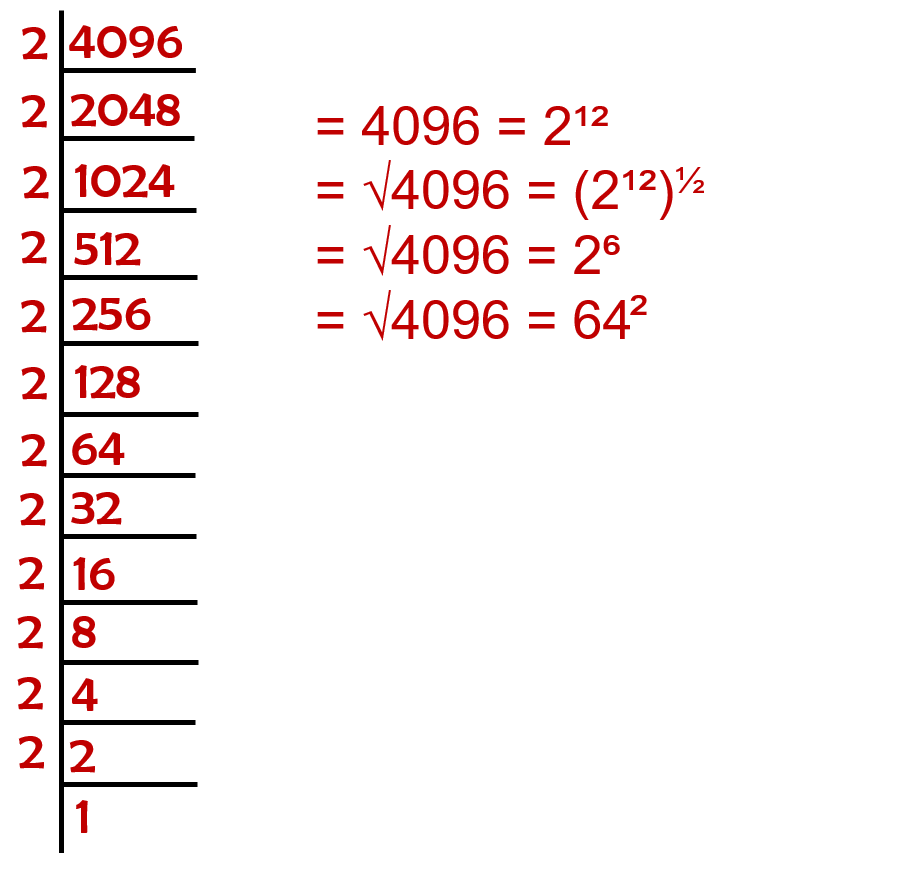v.vi.vii.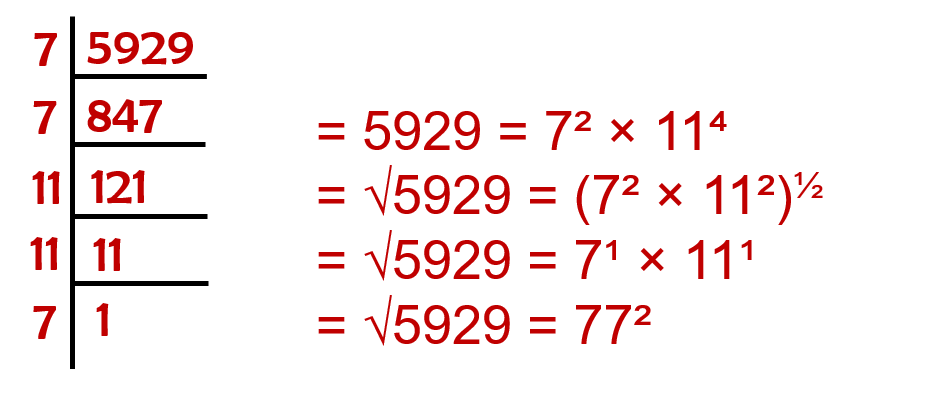viii.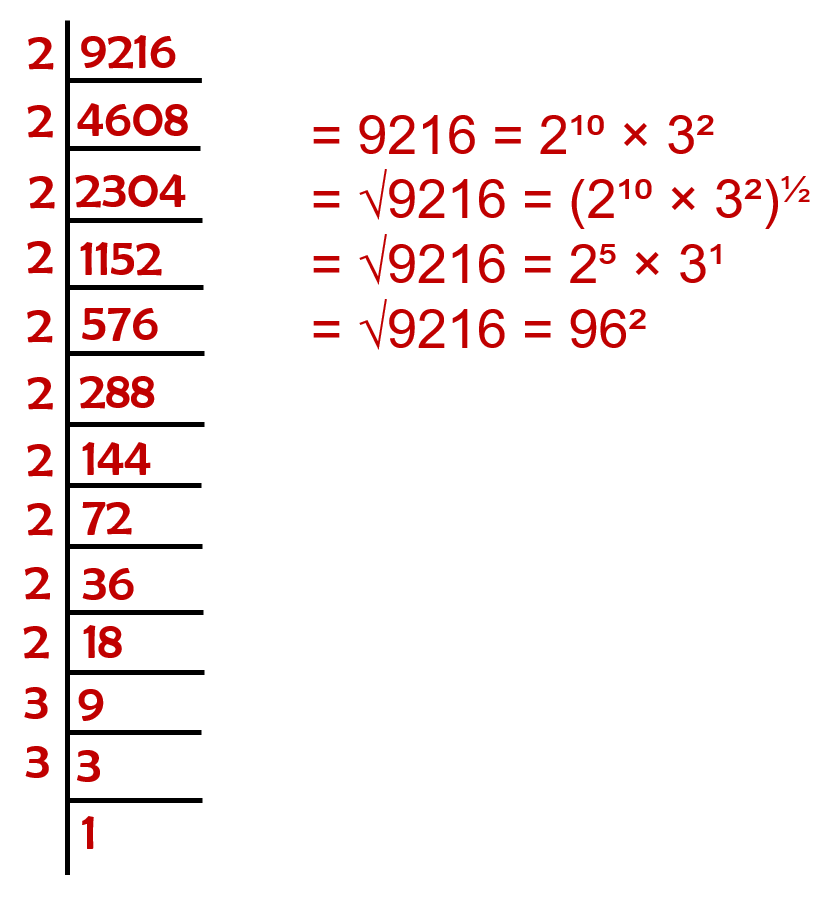ix.x.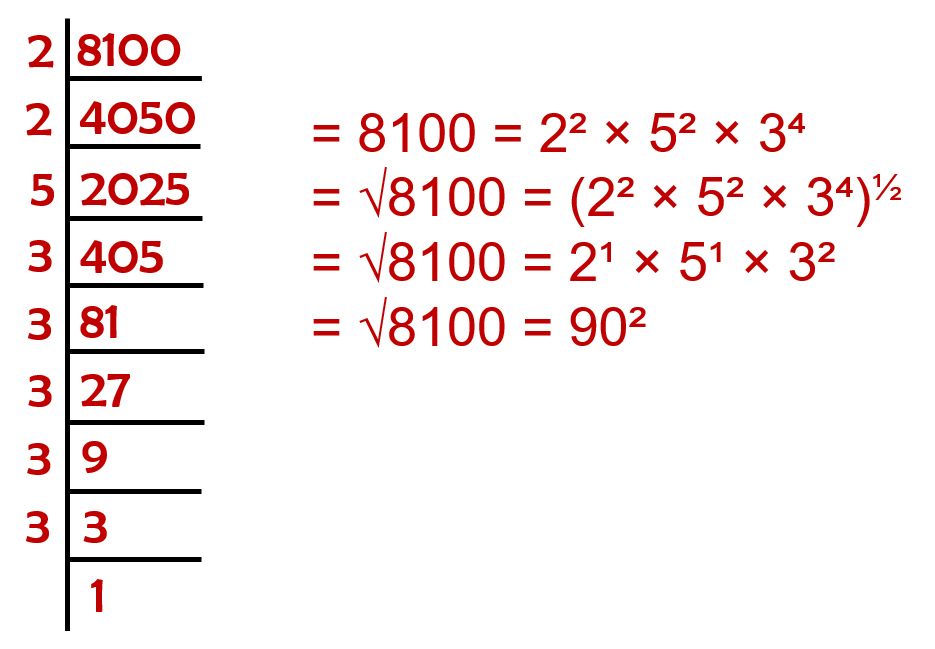Question 5: For each of the following numbers, find the smallest whole number by which it should be multiplied so as to get a perfect square number. Also find the square root of the square number so obtained.
i. 252
ii. 180
iii. 1008
iv. 2028
v. 1458
vi. 768

i.ii.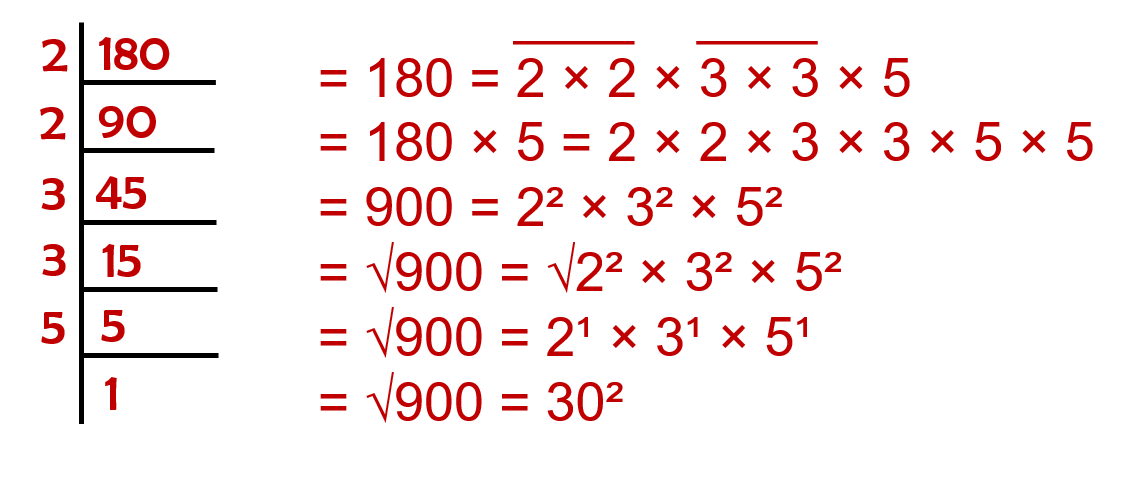iii.iv.v.vi.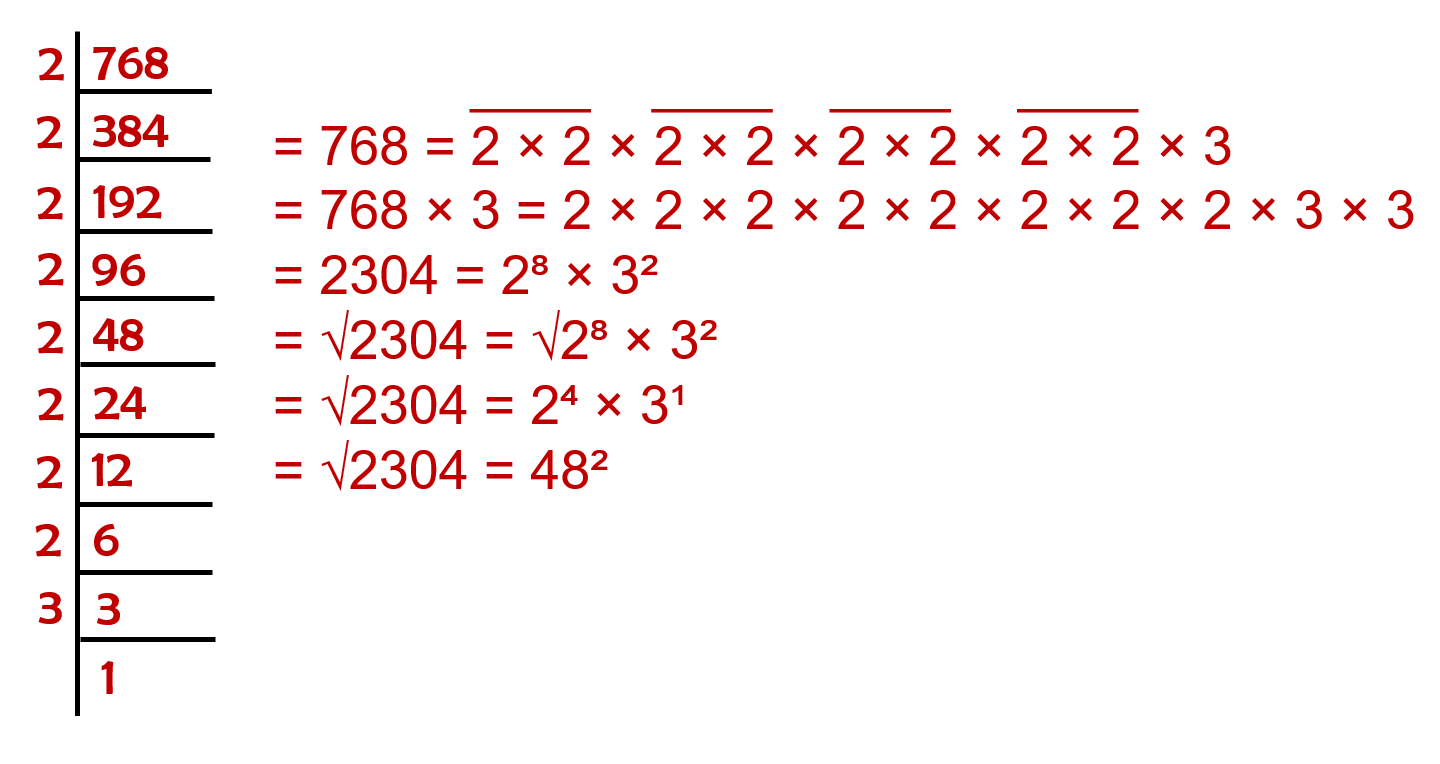Question 6: For each of the following numbers, find the smallest whole number by which it should be divided so as to get a perfect square. Also find the square root of the square number so obtained.
i. 252
ii. 2925
iii. 396
iv. 2645
v. 2800
vi. 1620

i.ii.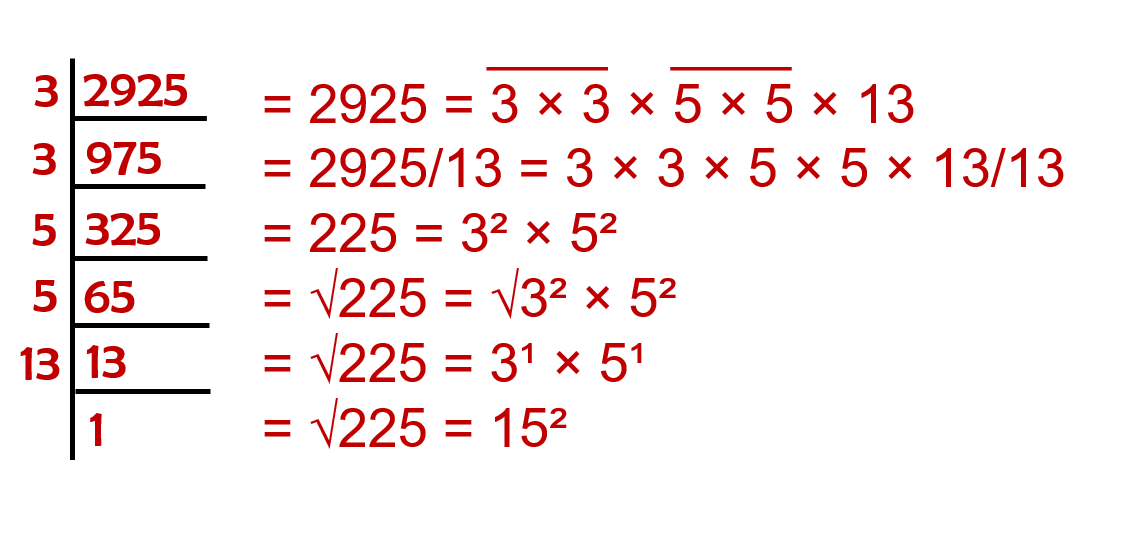iii.iv.v.vi.Question 7: The students of Class VIII of a school donated Rs 2401 in all, for Prime Minister’s National Relief Fund. Each student donated as many rupees as the number of students in the class. Find the number of students in the class.

Total amount donated = ₹2401
Let the number of students = x
Amount of donation by each student = ₹x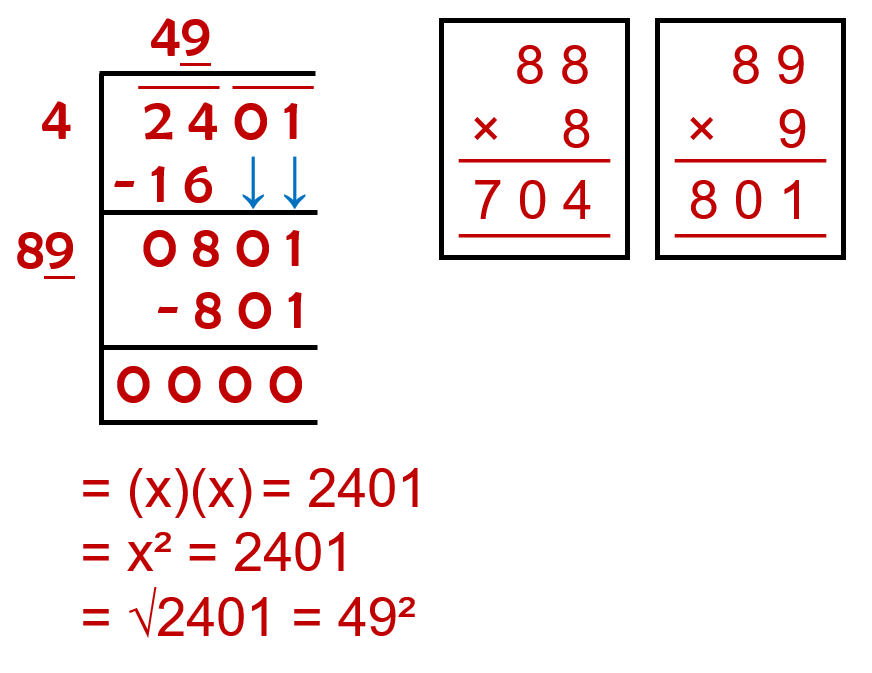Total students = 49
Amount donated by each student = ₹49

Therefore, the number of students are 49 and the money donated each one of them is ₹49.

Question 8: 2025 plants are to be planted in a garden in such a way that each row contains as many plants as the number of rows. Find the number of rows and the number of plants in each row.

Total plants to be planted = 2025
Let the number of rows = x
Let the number of plants in a row = xTotal rows = 45
Total plants in a row = 45

Therefore, the number of rows is 45 and number of plants to be planted in each row is 45.

Question 9: Find the smallest square number that is divisible by each of the numbers 4, 9 and 10.